Courses

# Formative Assessment - Data Handling Class 8 Notes | EduRev

## Mathematics (Maths) Class 8

Created by: Indu Gupta

## Class 8 : Formative Assessment - Data Handling Class 8 Notes | EduRev

The document Formative Assessment - Data Handling Class 8 Notes | EduRev is a part of the Class 8 Course Mathematics (Maths) Class 8.
All you need of Class 8 at this link: Class 8

Question 1: Complete the following crossword puzzle using the given directions for ACROSS [from left to right] and DOWN [from top to bottom].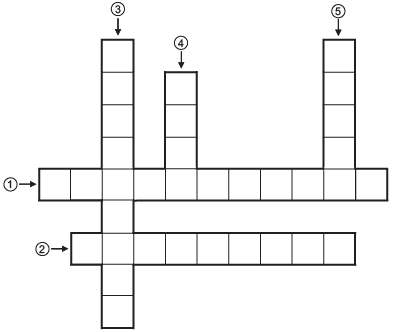Directions:
Accross: (1) __________ is the measure of the chance of the happening of an event.
(2) is the number of times a particular entry occurs. __________.

Down:
(3) __________ is a special type of bargraph in which the rectangles are having no  gap between them.
(4) The numerical information is called __________.
(5) The probability of a sure __________ is 1.

Question 2: Match the following:

 Column A Column B (a) Probability of a sure event (i) 0 (b) Probability of an impossible event. (ii) no (c) In a histogram there is ______ gap between the bars. (iii) 1 (d) In a bar graph there is _________ gap between the bars. (iv) equal

Question 3: Choose the appropriate answer:
(i) A coin tossed once; what is the  probability of getting a head?
(a) 1

(b) 2
(c) 1/2
(d) 0

(ii) In the interval (0–10), 10 is called the
(a) Lower limit
(b) upper limit
(c) range
(d) frequency

(iii) Tally marks are used to represent:
(a) Lower limits
(b) upper limits
(c) range
(d) frequency

(iv) The mid-value of the class interval is called class mark and it is equal to:
(a)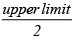(b)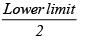(c)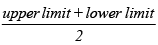(d)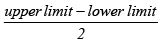Question 4: Answer the following questions:
(i) A die has six faces having dots, 1, 2, 3, 4, 5 and 6 respectively.

What is the sum of dots on two faces of a die ?
(ii) A die is thrown once. What is the probability of getting a prime-number?
(iii) What is the class size in the adjoining histogram?
(iv) What do we call a circle-graph?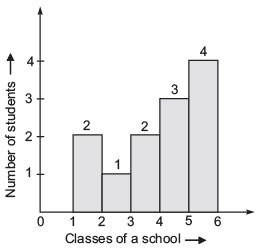1. (1) → PROBABILITY
(2) → FREQUENCY
(3) → HISTOGRAM
(4) → DATA

(5) → EVENT

2. (a) →  (iii)
(b) → (i)
(c) → (ii)
(d) → (iv)

3. (i) (c)
(ii) (b)
(iii) (d)
(iv) (c)

4. (i) 7
(ii) 1/2
(iii) 1
(iv) → piechart

,

,

,

,

,

,

,

,

,

,

,

,

,

,

,

,

,

,

,

,

,

;